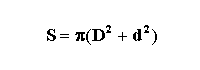Mathematics

Area/Volume of a Hollow Sphere

 Show figure Calculate:   Total Surface Area (S)  Volume (V) R =r  = D  =d  = To Memory 01 Memory 02 Memory 03 Memory 04 Memory 05 Memory 06 Replacing Adding Subtracting Multiplying Dividing
 Configurations Maximum of decimal places Unit of Measurement of Length: Impression Page Title (Optional) Comments to be printed (Optional)